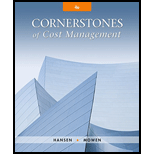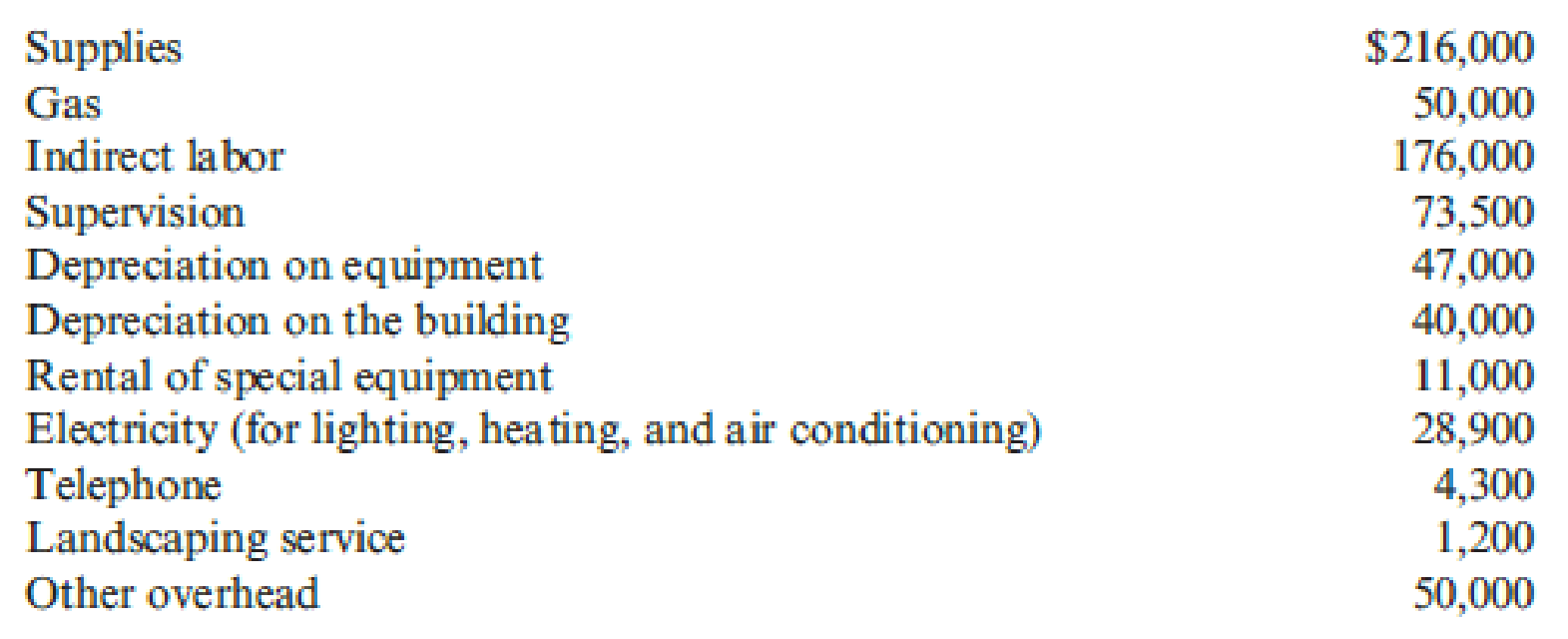### Cornerstones of Cost Management (C...

4th Edition
Don R. Hansen + 1 other
Publisher: Cengage Learning
ISBN: 9781305970663

#### Solutions

Chapter
Section### Cornerstones of Cost Management (C...

4th Edition
Don R. Hansen + 1 other
Publisher: Cengage Learning
ISBN: 9781305970663
Chapter 8, Problem 5CE
Textbook Problem
247 views

## Johnston Company cleans and applies powder coat paint to metal items on a job-order basis. Johnston has budgeted the following amounts for various overhead categories in the coming year.In the coming year, Johnston expects to powder coat 120,000 units. Each unit takes 1.3 direct labor hours. Johnston has found that supplies and gas (used to run the drying ovens—all units pass through the drying ovens after powder coat paint is applied) tend to vary with the number of units produced. All other overhead categories are considered to be fixed. (Round all overhead rates to the nearest cent.)Required: 1. Calculate the number of direct labor hours Johnston must budget for the coming year. Calculate the variable overhead rate. Calculate the total fixed overhead for the coming year. 2. Prepare an overhead budget for Johnston for the coming year. Show the total variable overhead, total fixed overhead, and total overhead. Calculate the fixed overhead rate and the total overhead rate (rounded to the nearest cent). 3. What if Johnston had expected to make 118,000 units next year? Assume that the variable overhead per unit does not change and the total fixed overhead amounts do not change. Calculate the new budgeted direct labor hours and prepare a new overhead budget. Calculate the fixed overhead rate and the total overhead rate (rounded to the nearest cent).

1.

To determine

Calculate the followings:

• Number of direct labor hours budgeted by J for the coming year.
• Total fixed overhead for the coming year.

### Explanation of Solution

Direct labor budget: A budget which shows the estimated amount payable to the labor in the exchange of their efforts during production cycle is known as direct labor budget.

Calculate the number of direct labor hours budgeted by J for the coming year:

Number of direct labor hours budgeted by J for the coming year}=(Budgeted units×Budgeted direct labor hours per unit)=(120,000×1.3)=156,000direct labor hours

Therefore the number of direct labor hours budgeted by J for the coming year is 156,000.

Step 1: Calculate the amount of variable overhead.

Amount of variable overhead=(Amount of supplies + Gas)=($216,000+$50,000)=\$266,000

Step 2: Calculate the variable overhead rate

2.

To determine

Calculate the followings:

3.

To determine

Calculate the followings:

• New budgeted direct labor hours.

### Still sussing out bartleby?

Check out a sample textbook solution.

See a sample solution

#### The Solution to Your Study Problems

Bartleby provides explanations to thousands of textbook problems written by our experts, many with advanced degrees!

Get Started

Find more solutions based on key concepts
Define an asset. What are the three characteristics of an asset?

Intermediate Accounting: Reporting And Analysis

RATIO CALCULATIONS Assume the following relationships for the Brauer Corp.: Sales/Total assets 1.5x Return on a...

Fundamentals of Financial Management, Concise Edition (with Thomson ONE - Business School Edition, 1 term (6 months) Printed Access Card) (MindTap Course List)

Give three examples of important trade-offs that you face in your life.

Principles of Microeconomics (MindTap Course List)

Explain why transferred-in costs are a special type of raw materials for the receiving department.

Managerial Accounting: The Cornerstone of Business Decision-Making

Define the term marketing

MKTG 12:STUDENT ED.-TEXT